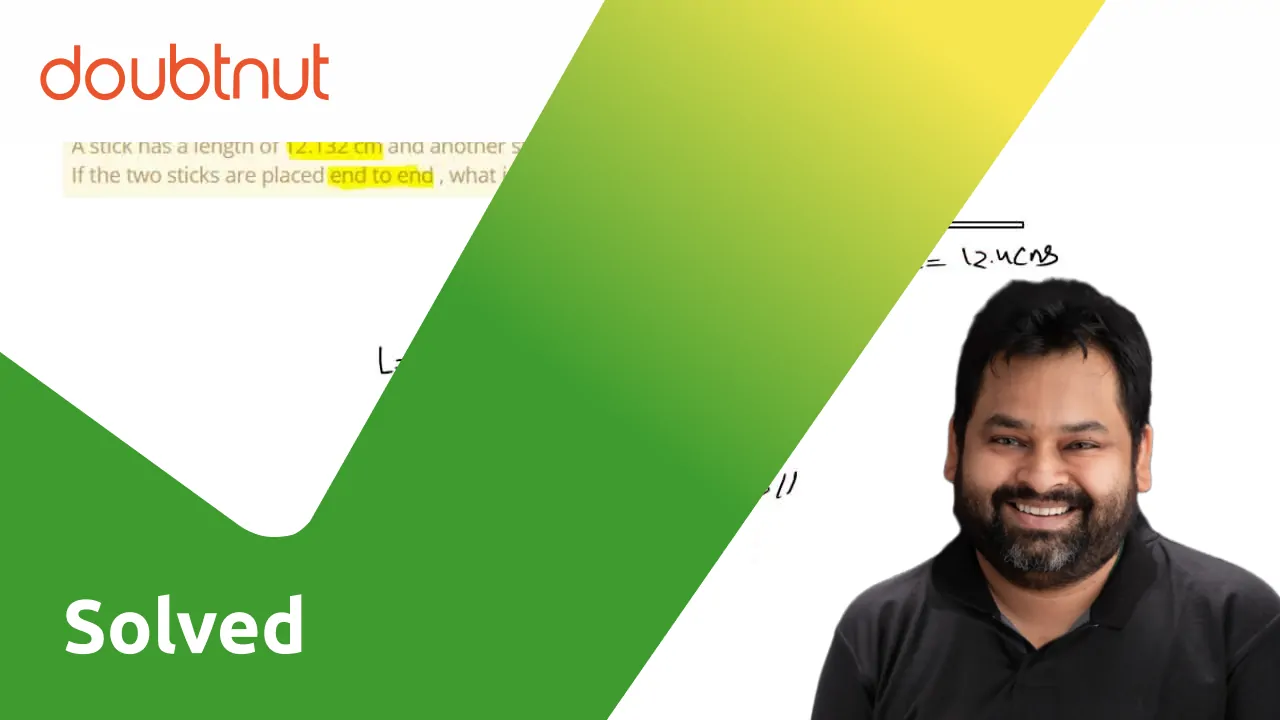Doubtnut App - Get 50 Lakh+ video solutions better than GoogleInstall Now

# A stick has a length of 12.132 cm and another stick has a length of 12.4 cm. If the two sticks are placed end to end , what is their total length ?Solution in Telugu

Text Solution
Verified by Experts

## (a) Let lengths of the sticks are named as l1=12.132cm,l2=12.4cm Here l2 has one decimal place and l1 has to be rounded off to have only two decimal places l1+l2=12.13+12.4=24.53 This is to be rounded off to have one decimal place only ∴ The total length is 24.5cm (b) l1=12.132,l2=12.4 l1−l2=12.4−12.132 Here 12.4 has only decimal place and hence 12.132 shoudl have only two decimal places. ∴l1−l2=12.4−12.13=0.27 This should be rouned off to have only one decimal place ∴l1−l2=0.3 Hence difference of their lengths is 0.3cm